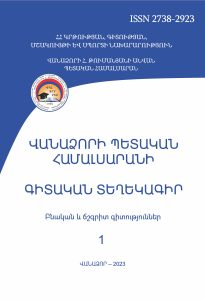# The Assignment Problem and the Program for its Solution

Scientific Proceedings of  Vanadzor State University Natural and Exact Sciences (ISSN 2738-2923)

2023 vol 1

The Assignment Problem and the Program for its Solution

Ruzanna Mazmanyan

SummaryKey words: linear programming, programming language, Hungarian method, algorithm, parameter, optimal solution, interval

The article deals with one of the fundamental problems of linear programming – the assignment problem. It considers the software implementation of solving the assignment problem.

The specific features of assignment problems gave rise to an effective Hungarian method for their solution. The main idea of the Hungarian method is to move from the original square cost matrix to an equivalent matrix with non-negative entries and a system of independent zeros, no two of which belong to the same row or the same column.

The algorithm is based on two ideas:

• if one and the same number is subtracted from all elements of a certain row or column, such that all elements of the matrix remain non-negative, then the optimal solution will not change,
• if there is a zero cost solution, then it is optimal.

The program is written with the object-oriented programming language C# and runs in C# graphics mode.

The program that implements the solution of the problem at the beginning requires the input of initial data, forms a square matrix of prices in the graphical mode, and then, by performing a sequence of steps of the Hungarian method algorithm, the final solution of the problem is achieved.

According to the compiled square price matrix, the algorithm finds the optimal distribution of work among performers, so that all tasks are distributed and each performer gets exactly one task. The program also calculates the total cost necessary to fulfill the entire work.

In the graphical mode of the C# programming language, a step-by-step implementation of solving the assignment problem using the Hungarian method is demonstrated.

PDF